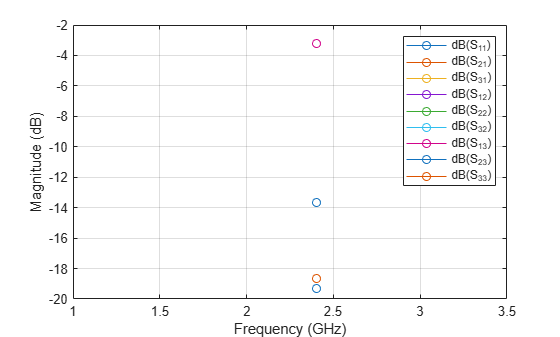sparameters

Calculate S-parameters for RF PCB objects

Description

sobj = sparameters(rfpcbobj,freq) calculates the S-parameters for the RF PCB object rfpcbobj over the specified frequency values.

example

sobj = sparameters(___,Z0) calculates the S-parameters for the reference impedance Z0.

example

sobj = sparameters(___,Name=Value) calculates S-parameters using one or more name-value arguments in addition to any of the input argument combinations in previous syntaxes

sobj = sparameters(data,freq) creates an S-parameter object from the S-parameter data provided in data over the specified frequencies values.

sobj = sparameters(data,freq,Z0) creates an S-parameter object for the reference impedance Z0.

sobj = sparameters(filename) creates an S-parameter object from the data provided in the Touchstone file specified in filename.

Examples

collapse all

Create a Wilkinson power splitter object.

rfpcbobj = wilkinsonSplitter;

Calculate the S-parameters for the Wilkinson power splitter at 2.4 GHz with the reference impedance of 50 ohms.

Sobj = sparameters(wilkinsonSplitter,2.4e9,50);

Plot the S-parameters using the rfplot function.

rfplot(Sobj)Design a microstrip transmission line at 3 GHz with 75 ohms impedance.

m = microstripLine(Length=0.0379,Width=0.0027,Height=0.0016,GroundPlaneWidth=0.0133);

Create a right angle bend with length equal to half the length of the transmission line and width equal to the width of the transmission line.

layer2d = bendRightAngle( Length=[m.Length/2 m.Length/2],...
Width=[m.Width m.Width]);

Convert the right angle bend to a 3-D component.

robj = pcbComponent(layer2d);

Add thickness and substrate layers to the board.

robj.BoardThickness = m.Substrate.Thickness;
robj.Layers{2} = m.Substrate;

Define frequency points to calculate the S-parameters.

freq = (1:2:40)*100e6;

Calculate the S-parameters of the right angle bend using the behavioral model.

Sckt1 = sparameters(robj,freq,Behavioral=true);

Calculate the S-parameters of the right angle bend using the electromagnetic solver.

Sem1 = sparameters(robj,freq);

Plot the S-parameter data using the rfplot function.

rfplot(Sckt1,'abs','o')
hold on
rfplot(Sem1,'abs','s')Input Arguments

collapse all

Input object, specified as a RF PCB object. You can specify either a PCB component, a microstrip bend, or a trace. For complete list of PCB components, microstrip bends, and traces, see PCB Components Catalog and Custom Geometry and PCB Fabrication.

S-parameter data, specified as an array of complex numbers of the size N-by-N-by-K, where K represents number of frequency points.

S-parameter frequencies, specified as a scalar or vector of positive real numbers in the ascending order.

Reference impedance in ohms, specified as a positive real scalar.

Name of the Touchstone file containing network parameter data, specified as a character vector or string scalar. If the file is in the current folder or in a folder on the MATLAB® path, specify the file name. If the file is not in the current folder or in a folder on the MATLAB path, then specify the full or relative path name.

Example: sobj = sparameters('defaultbandpass.s2p');

Name-Value Arguments

Specify optional comma-separated pairs of Name,Value arguments. Name is the argument name and Value is the corresponding value. Name must appear inside quotes. You can specify several name and value pair arguments in any order as Name1,Value1,...,NameN,ValueN.

Example: Sobj = sparameters(robj,freq,Behavioral=true)

Behavioral model of an RF PCB component and bend, specified as true (1) or false (0). You can compute the behavioral model for these rfpcb objects:

 Bends Traces Transmission line objects Inductor spiralInductor Capacitor interdigitalCapacitor

Note

• Before using the sparameters function to calculate S-parameters for bends and traces, convert bends and traces to PCB components using the pcbComponent function.

• The sparameters function does not support using the behavioral model argument for:

• Objects with unequal widths like bendRightAngle, bendCurved, and bendMitered

• Asymmetric tee and cross-junction traces

Example: Sobj = sparameters(microstripline,freq,Behavioral = true)

Output Arguments

collapse all

S-parameters, returned as an object with the following properties:

• NumPorts — Number of ports, N, returned as an integer. The function calculates this value automatically when you create the object.

• Frequencies — S-parameter frequency, returned as a scalar or row vector of length, K, in the ascending order. The function sets this property from the filename or freq input arguments.

• Parameters — S-parameters, returned as an N-by-N-by-K array of complex numbers. The function sets this property from the filename or data input arguments.

• Impedance — Reference impedance in ohms, returned as a positive real scalar. The function sets this property from the filename or Z0 input arguments. If you do not provide reference impedance,, the function uses a default value of 50.